# Sets + intersection of sets - math problems

#### Number of problems found: 58

• Subtracting sets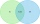For two sets K, L is true: K has 30 elements, L has 27 elements and the set L - K has 22 elements. How many elements does the set K - L have?
• Two sets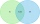Suppose Set B contains 69 elements and the total number elements in either Set A or Set B is 124. If the Sets A and B have 29 elements in common, how many elements are contained in set A?
• DisjointHow many elements have union and intersection of two disjoint sets when the first have 1 and secodn 8 elements.
• Operations with sets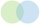The set B - A has twice as fewer elements than the set A - B and four times fewer elements than the set A ∩ B. How many times more elements does the set A have than the set B?
• The triangleThe triangle is given by three vertices: A [0.0] B [-4.2] C [-6.0] Calculate V (intersection of heights), T (center of gravity), O - center of a circle circumscribed
• Probability of intersectionThree students have a probability of 0.7,0.5 and 0.4 to graduated from university respectively. What is the probability that at least one of them will be graduated?
• Coordinates of the intersection of the diagonals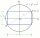In the rectangular coordinate system, a rectangle ABCD is drawn. The vertices of the rectangle are determined by these coordinates A = (2.2) B = (8.2) C = (8.6) D = (2.6) Find the coordinates of the intersection of the diagonals of the ABCD rectangle
• Intersect and conjuctionLet U={1,2,3,4,5,6} A={1,3,5} B={2,4,6} C={3,6} Find the following. 1. )AUB 2. )A'UB'
• Intersections 3Find the intersections of the circles x2 + y2 + 6 x - 10 y + 9 = 0 and x2 + y2 + 18 x + 4 y + 21 = 0
• Marriage sttusIn our city, there are 3/5 of the women married to 2/3 of the men. Find what part of the population is free.
• Dices throwsWhat is the probability that the two throws of the dice: a) Six falls even once b) Six will fall at least once
• Glasses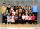Imagine a set of students in your class (number of students: 19), who wears glasses. How much minimum and maximum element may contain this set.
• Trousers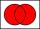In the class was 12 students. Nine students wearing trousers and turtleneck eight. How many students worn trousers with a turtleneck?
• Perpendicular projectionDetermine the distance of a point B[1, -3] from the perpendicular projection of a point A[3, -2] on a straight line 2 x + y + 1 = 0.
• Line intersect segment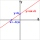Decide whether the line p : x + 2 y - 7 = 0 intersects the line segment given by points A[1, 1] and B[5, 3]
• Left handedIt is known that 25% of the population is left-handed. What is the probability that there is a maximum of three left-handers at a seminar where there are 30 participants?
• Fall sum or sameFind the probability that if you roll two dice, it will fall the sum of 10, or the same number will fall on both dice.
• CoordinatesDetermine the coordinates of the vertices and the content of the parallelogram, the two sides of which lie on the lines 8x + 3y + 1 = 0, 2x + y-1 = 0 and the diagonal on the line 3x + 2y + 3 = 0
• Wafers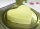They used 200 pieces to make wafers. 157 topped with caramel topping and 100 with sugar topping. How many wafers did both toppings have?
• Language coursesOf the company's 60 employees, 28 attend an English course, 17 take a German course, and 20 do not attend any of these courses. How many employees attend both courses?

Do you have an interesting mathematical word problem that you can't solve it? Submit a math problem, and we can try to solve it.

We will send a solution to your e-mail address. Solved examples are also published here. Please enter the e-mail correctly and check whether you don't have a full mailbox.

Please do not submit problems from current active competitions such as Mathematical Olympiad, correspondence seminars etc...

Sets - math word problems. Intersection of sets - math word problems.